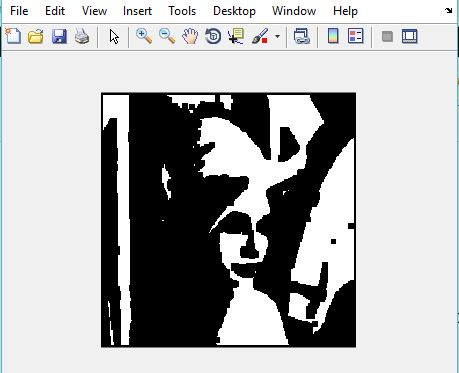Related Articles
Matlab | Erosion of an Image
• Last Updated : 07 Jun, 2019

Morphology is known as the broad set of image processing operations that process images based on the shapes. It is also known as a tool used for extracting image components that are useful in representation and description of region shape.

The basic morphological operations are:
1. Erosion
2. Dilation

In this article, we will be discussing Erosion.

### Erosion:

• Erosion shrink-ens the image pixels i.e. it is used for shrinking of element A by using element B.
• Erosion removes pixels on object boundaries.:
• The value of the output pixel is the minimum value of all the pixels in the neighborhood. A pixel is set to 0 if any of the neighboring pixels have the value 0.

Approach:

1. Read the RGB image.
2. Using function `im2bw()`, convert the RGB image to binary image.
3. Create a structuring element or you can use any predefined mask eg. fspecial(‘sobel’).
4. Store the number of rows and columns in an array and loop through it.
5. Create a zero matrix of the size same as of the size of our image.
6. Leaving the boundary pixels start moving the structuring element on the image and start comparing the pixel with the pixels present in the neighborhood.
7. If the value of neighborhood pixel is 0, then change the value of that pixel to 0.

Below is the Matlab code for Erosion:

 `% read image``I=imread(``'lenna.png'``);   `` ` `% convert to binary  ``I=im2bw(I); `` ` `% create structuring element              ``se=ones(5, 5);`` ` `% store number of rows in P and number of columns in Q.            ``[P, Q]=size(se); `` ` `% create a zero matrix of size I.        ``In=zeros(size(I, 1), size(I, 2)); `` ` `for` `i=ceil(P/2):size(I, 1)-floor(P/2)``    ``for` `j=ceil(Q/2):size(I, 2)-floor(Q/2)`` ` `        ``% take all the neighbourhoods.``        ``on=I(i-floor(P/2):i+floor(P/2), j-floor(Q/2):j+floor(Q/2)); `` ` `        ``% take logical se``        ``nh=on(logical(se)); ``       ` `        ``% compare and take minimum value of the neighbor ``        ``% and set the pixel value to that minimum value. ``        ``In(i, j)=min(nh(:));      ``    ``end``end`` ` `imshow(In);`

Input Image:Output Image:Attention reader! Don’t stop learning now. Get hold of all the important CS Theory concepts for SDE interviews with the CS Theory Course at a student-friendly price and become industry ready.

My Personal Notes arrow_drop_up